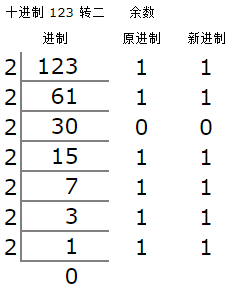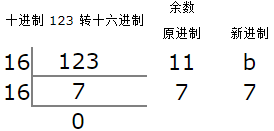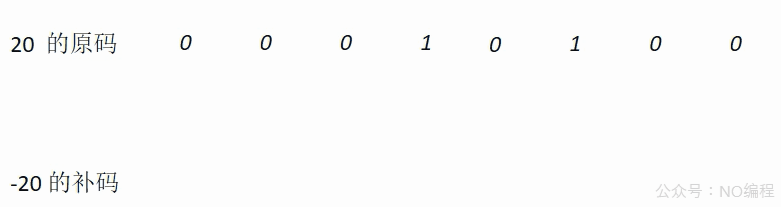# 三分钟熟悉进制转换与位运算

## 进制转换

1. 将十进制数 123 转换为二进制。1. 将十进制数 123 转换为十六进制。N 进制转为十进制可以通过“按位权展开法”来转换，即在 N 进制中，每个位置的数字乘以进制的基数为底的所处位置序号(从 0 开始)为指数的整数次幂，然后相加。例如：

1. 将二进制数 1111011 转换为十进制。

1 * 2^6 + 1 * 2^5 + 1 * 2^4 + 1 * 2^3 + 0 * 2^2 + 1 * 2^1 + 1 * 2^0
= 1 * 64 + 1 * 32 + 1 * 16 + 1 * 8 + 0 * 4 + 1 * 2 + 1 * 1
= 123

1. 将十六进制数 7b 转换为十进制

7 * 16^1 + b * 16^0
= 7 * 16 + 11 * 1
= 123

### 负数的二进制

-11 = 10001011(原码) = 11110100(反码)。

### 一种简单方式算出补码

1. 将对应的正数原码从最低比特位向高比特位查找。
2. 若该比特位为 0，补码对应比特位填 0，继续向高比特位查找。
3. 若找到第一个为 1 的比特位，将补码对应比特位填 1。
4. 然后，将其余未转换的比特位全部取反。### Java 内置的进制转换 API

JDK 的 Integer 和 Long 类提供了常用的进制相互转换方法。

10进制 → 2进制 toBinaryString(int i) toBinaryString(long i)
10进制 → 8进制 toOctalString(int i) toOctalString(long i)
10进制 → 16进制 toHexString(int i) toHexString(long i)
10进制 → n 进制 toString(int i, int radix) toString(long i, int radix)
n 进制 → 10进制 valueOf(String s, int radix)
parseInt(String s, int radix)
valueOf(String s, int radix)
parseLong(String s, int radix)
System.out.println(Integer.toBinaryString(13)); // 十转二，结果为 1101
System.out.println(Integer.toOctalString(13));  // 十转八，结果为 15
System.out.println(Integer.toHexString(13));    // 十转十六，结果为 d
System.out.println(Integer.toString(13, 5));    // 十转五，结果为 23
System.out.println(Integer.valueOf("23", 5));   // 五转十，结果为 13


String fullStr = StringUtils.leftPad("1010", 32, "0"); // 对二进制 1010 位数补齐
System.out.println(fullStr); // 输出结果：00000000000000000000000000001010


### Java 中进制前缀

Java 对二进制、八进制、十六进制提供了字面量前缀的表现形式，可以直接使用这几种进制形式计算或赋值，例如：

// 都表示十进制的 10
int i1 = 10; 	 // 十进制，没有前缀
int i2 = 0b1010; // 二进制，前缀   0b(Java 7 或更高版本)
int i3 = 012; 	 // 八进制，前缀   0
int i4 = 0xA; 	 // 十六进制，前缀 0x

System.out.println(i2 + 1);
System.out.println(i3 + 1);
System.out.println(i4 + 1);


## 位运算

### & (按位与)### | (按位或)### ~ (按位非)### ^ (按位异或)### << (左移)### >> (右移)### >>> (无符号右移)### 应用示例

1. m * 2^n 或 m / 2^n
System.out.println(2 << 1); // 2 * 2^1 = 4 ，相当于乘以 2^1
System.out.println(3 >> 1); // 3 / 2^1 = 1 , 相当于除以 2^1 向下取整

1. 奇数偶数判断
if(m & 1 == 1) {
System.out.println("奇数");
} else {
System.out.println("偶数");
}

1. 随机概率
// lr = 随机数;
if ((lr & 0x3) == 0) {
// 有 1/4 的概率执行该代码块
}


posted @ 2021-02-24 10:43  陆十三  阅读(340)  评论(2编辑  收藏